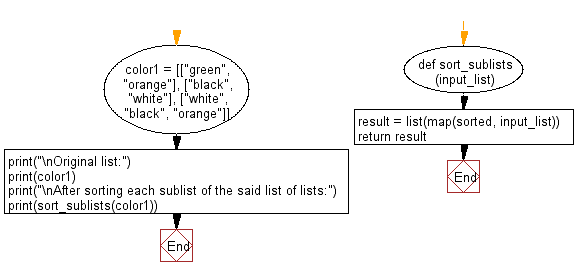﻿ Python: Sort each sublist of strings in a given list of lists - w3resource# Python: Sort each sublist of strings in a given list of lists

## Python List: Exercise - 95 with Solution

Write a Python program to sort each sublist of strings in a given list of lists.

Sample Solution:

Python Code:

``````def sort_sublists(input_list):
result = list(map(sorted, input_list))
return result
color1 = [["green", "orange"], ["black", "white"], ["white", "black", "orange"]]
print("\nOriginal list:")
print(color1)
print("\nAfter sorting each sublist of the said list of lists:")
print(sort_sublists(color1))
```
```

Sample Output:

```Original list:
[['green', 'orange'], ['black', 'white'], ['white', 'black', 'orange']]

After sorting each sublist of the said list of lists:
[['green', 'orange'], ['black', 'white'], ['black', 'orange', 'white']]
```

Flowchart:## Visualize Python code execution:

The following tool visualize what the computer is doing step-by-step as it executes the said program:

Python Code Editor:

Have another way to solve this solution? Contribute your code (and comments) through Disqus.

What is the difficulty level of this exercise?

Test your Python skills with w3resource's quiz

﻿

## Python: Tips of the Day

Floor Division:

When we speak of division we normally mean (/) float division operator, this will give a precise result in float format with decimals.

For a rounded integer result there is (//) floor division operator in Python. Floor division will only give integer results that are round numbers.

```print(1000 // 300)
print(1000 / 300)```

Output:

```3
3.3333333333333335```